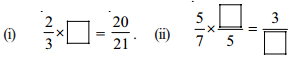# AP Board 7th Class Maths Solutions Chapter 2 Fractions, Decimals and Rational Numbers Ex 3

AP State Syllabus AP Board 7th Class Maths Solutions Chapter 2 Fractions, Decimals and Rational Numbers Ex 3 Textbook Questions and Answers.

## AP State Syllabus 7th Class Maths Solutions 2nd Lesson Fractions, Decimals and Rational Numbers Exercise 3Question 1.
Find each of the following products.
(i) $$\frac{5}{6} \times \frac{7}{11}$$
(ii) $$6 \times \frac{1}{5}$$
(iii) $$2 \frac{1}{3} \times 3 \frac{1}{5}$$
Solution: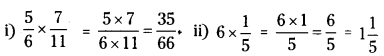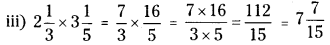Question 2.
Multiply and reduce to lowest form.
(i) $$\frac{2}{3} \times 5 \frac{1}{5}$$
(ii) $$\frac{2}{7} \times \frac{1}{3}$$
(iii) $$\frac{9}{3} \times \frac{5}{5}$$
Solution: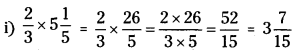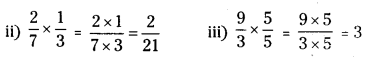Question 3.
Which one is greater’?
(i) $$\frac{2}{5} \text { of } \frac{4}{7} \text { or } \frac{3}{4} \text { of } \frac{1}{2}$$
(ii) $$\frac{1}{2} \text { of } \frac{4}{7} \text { or } \frac{2}{3} \text { of } \frac{3}{7}$$
Solution:
(i) $$\frac{2}{5} \text { of } \frac{4}{7} \text { or } \frac{3}{4} \text { of } \frac{1}{2}$$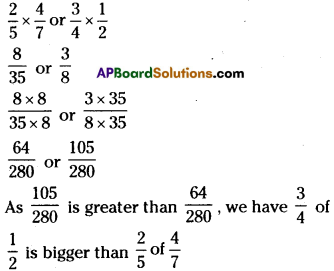(ii) $$\frac{1}{2} \text { of } \frac{4}{7} \text { or } \frac{2}{3} \text { of } \frac{3}{7}$$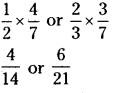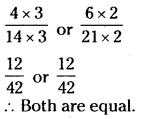Question 4.
Rehana works 2$$\frac{1}{2}$$ hours each day on her embroidery. She completes the work in 7 days.
How many hours did she take to complete her work?
Solution:
Number of hours Rehana worked each day = 2$$\frac{1}{2}$$ hrs
Number of days Rehana worked on embroidery = 7 days
Total time worked = 2½ x 7 = $$\frac{5}{2}$$ x 7 = $$\frac{5 \times 7}{2}=\frac{35}{2}$$ = = 17$$\frac{1}{2}$$ hrs.

Question 5.
A truck runs 8 km using 1 litre of petrol. How much distance will it cover using 10$$\frac{2}{3}$$ litres of petrol?
Solution:
bistance covered wIth 1 litre of petrol 8 km
∴ Distance covered for 10$$\frac{2}{3}$$ litres petrol = 10$$\frac{2}{3}$$ x 8
= $$\frac{32}{3} \times 8=\frac{32 \times 8}{3}=\frac{256}{3}=85 \frac{1}{3}$$km.Question 6.
Raja walks 1$$\frac{1}{2}$$ meters in 1 second. How much distance will he walk in 15 minutes?
Solution:
Distance covered by Raja in 1 second = 1$$\frac{1}{2}$$ m
Distance covered by Raja in 15 minutes 15 x 60 x 1$$\frac{1}{2}$$
= 900 x $$\frac{3}{2}$$ = $$\frac{900\times 3}{21}$$ =1350 mQuestion 7.
Provide the number in the box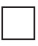to make the statement true.